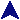Serial IntegrationSome PVM Examples```#include <stdio.h>
#include <stdlib.h>
#include <math.h>
#include "func.h"

/*
* Find the area to the tolerance.
*/
static double find(double x1, double y1, double x2, double y2, double tol)
{
/* Compute the midpoint from the funcion. */
double midx = (x1 + x2) / 2;
double midy = f(midx);

/* Estimate the midpoint from the y value. */
double midest = (y1 + y2) / 2;

//printf("FRED: %g %g %g %g %g %g\n", x1, y1, x2, y2, midx, midy);

/* See if we're getting close. */
if(fabs(midy - midest) <= tol)
/* Will do.  Compute the area using the midy, since we
found it.  Two trapazoids algebraically simplified. */
return 0.25*(x2-x1)*(y1 + y2 + 2*midy);
else
/* Subdivide and try again. */
return find(x1, y1, midx, midy, tol) +
find(midx, midy, x2, y2, tol);
}

/*
* Simple numerical integration.  Function with double(double) signature
* is linked in.
*/
int main(int argc, char **argv)
{
/* Fine argument whine. */
if(argc < 5) {
printf("Usage: %s startx endx tol nreg funcargs*\n", argv);
exit(2);
}

/* Get the args. */
double start = atof(argv);
double end = atof(argv);
double tol = atof(argv);
int nreg = atoi(argv);

/* Sanity. */
if(tol < 0.0000000001) {
printf("Tolerance to too small or negative.\n");
exit(3);
}
if(nreg < 1) {
printf("Number of regions is nonpositive.\n");
exit(4);
}
if(end < start) {
double t = start;
start = end;
end = t;
}

/* Initialize the function. */
finit(argc - 5, argv + 5);

/* Divide into to nreg regions, then find each to tolerance. */
double x = start;
double y = f(x);
double inc = (end - start) / nreg;
double tot = 0.0;
while(nreg--) {
double newx = nreg ? x + inc : end;
double newy = f(newx);
tot += find(x, y, newx, newy, tol);
x = newx;
y = newy;
}

printf("Integral from %g to %g = %g (tol %g)\n", start, end, tot, tol);
}
```